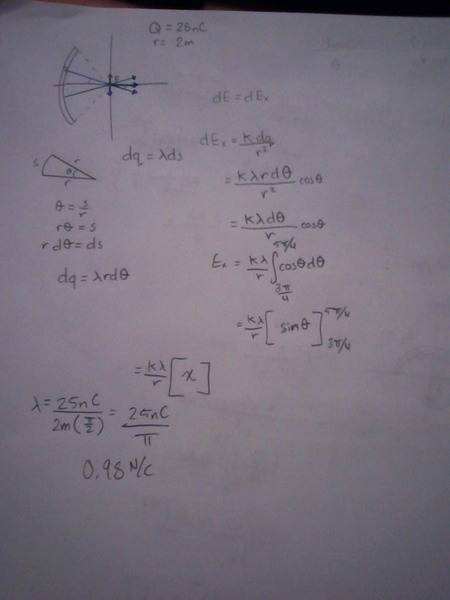# Find electric field on a point due to a charged arc.

## Homework Statement

A charge of 25nC is uniformly distributed along a circular arc (radius = 2.0 m) that is subtended by a 90-degree angle. What is the magnitude of the electric field at the center of the circle along which the arc lies?

I'm not getting the right answer however, so if someone would be able to help me solve this question and point out what I'm doing wrong I"d be very appreciative.

E = k*Q/r^2
θ=s/r
dq = λ ds

## The Attempt at a SolutionThe limits of integration should be $\frac{-\pi}{4}$ to $\frac{\pi}{4}$.
The arc length is $\frac{(2)(\pi)(r)}{4}$.
The limits of integration should be $\frac{-\pi}{4}$ to $\frac{\pi}{4}$.